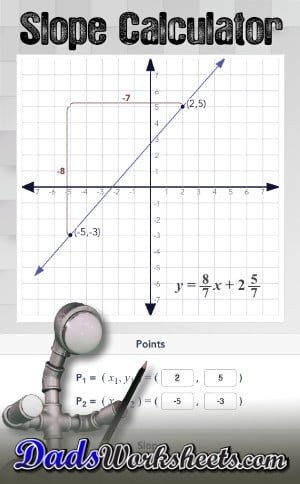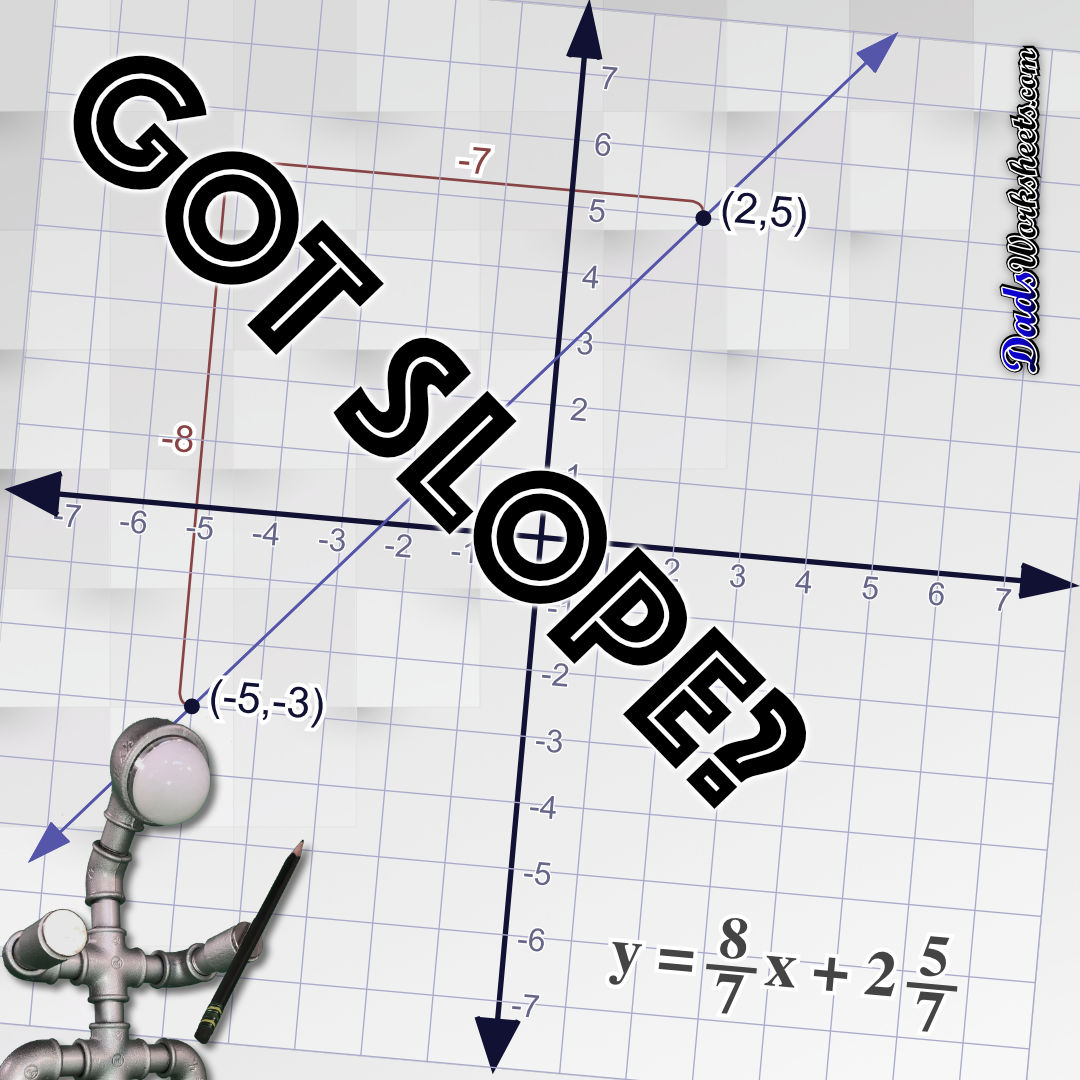# Slope Calculator

## Using the Slope Calculator

This slope calculator takes two points and then uses the slope formula to calculate the slope of a line defined by those two points, and then the y intercept. The slope and the intercept are then combined to provide the equation of the line in slope intercept form ("y=mx+b"). A graph of the line is drawn on a coordinate plane, along with the slope intercept equation.

The slope calculator updates the graph and the equation automatically when you enter new values for the points. The calculator also allows direct entry of the rise or run values, or a decimal value for m. The calculator will automatically create the correct decimal or fraction components for whatever you enter.

You may also calculate the equation for a line by changing the slope independently (either as a slope fraction or a slope decimal), or by entering a new y intercept. If a new slope is entered, the slope calculator will move one of the points so that the equation matches the new line. If a new y intercept is entered, the slope will remain the same but the calculator will move the two points to shift the line to match the new y intercept.

## What is the Slope of a Line?

The slope of a line is a mathematical measurement of how steep a line drawn on a graph appears, and this value is usually shown as the variable m in an equation in slope intercept form, y=mx+b.

Slope is defined as the ratio of vertical (y-axis) change over a given amount of horizontal (x-axis) change, often remembered more simply as a fraction describing rise over run or the rate of change. This slope calculator provides this ratio both as a fraction and a decimal, but shows the slope as a fraction in the calculator graph.

If a line is sloping up and to the right, it is rising as you look left-to-right across the x-axis. The rise in this case is positive, and such a line will have a positive slope.

If a line is sloping down and to the right, it is falling as you look left-to-right across the x-axis. The rise in this case is negative (the line is "falling"), and such a line will have a negative slope.

## The Slope Intercept Form

When we're dealing with an equation that describe lines (i.e., a linear equation), we typically put the equations into a form called slope intercept form that looks like this…

## Slope Intercept Form

y = mx + b

An equation in this form describes how the y coordinate for a point on the line is calculated given an x coordinate. The slope calculator takes the points you provide and then calculates the slope and the y intercept as described below. These values are combined and the equation of the line is shown in the calculator graph area.

## How to Find the Slope of a Line

If you have two points, they define a line on a Cartesian coordinate plane, and you can use those points to calculate the slope of the line. This slope calculator does exactly that using the formula below…

## Slope Equation

m =
rise
run
=
y2-y1
x2-x1

By starting with two points (x1,y1) and (x2,y2), the slope calculator substitutes the values into this equation to calculate the "rise" on the top and the "run" on the bottom. When choosing between your two points, it doesn't matter which point is used as (x1,y1) or (x2,y2), but it is very important that you consistently use the respective individual coordinates within each point. For example, if you choose one point such as (5, 6), be sure to use 6 (the y coordinate) as the first term of the "rise" subtraction on the top of the equation, and 6 as the first term of the "run" subtraction on the bottom of the equation. Mixing the individual coordinates between points or thinking that there's some specific reason to choose one point as (x1,y1) are common mistakes when calculating slope. When in doubt, check your answer with this slope calculator and you'll see it's a lot easier than it seems.

## What is the Slope of a Horizontal Line?

The slope of a horizontal line is equal to zero. In the slope formula above, the top component of the slope ratio shows the vertical change between two points on the line. Because every point on a horizontal line will have the same y-axis coordinate, the numerator in this slope fraction will always be zero, and therefore the calculated slope will also always be zero.

The slope calculator will calculate the equation of the line without the first term, effectively reducing the y=mx+b equation to the form y=b, reflecting that the calculated y coordinate is constant for any given x coordinate.

## What is the Slope of a Vertical Line?

Like the slope of a horizontal line, the slope of a vertical line is special. Again, referring to the slope equation, consider the way coordinates change as you travel up and down a vertical line. In the case of a vertical line, the x-axis coordinate will never change for any given y-axis coordinate. Because of this, the change in the x-axis represented as the bottom component of the slope ratio is zero.

There's a problem here. The slope equation divides by this change in x value result, and division by zero is not allowed. As a result, the slope of a vertical line is undefined, and you can readily see you cannot calculate y values in terms of x values using an equation in the y=mx+b slope intercept form because the m value for slope is undefined, making the whole equation undefined.

Simply put, there is not equivalent slope intercept form equation for a vertical line, so we need something else.

The equation for a vertical line is transformed by the slope calculator to the form x=c, where c represents a constant x value that defines the line for every possible y coordinate.

## How to Find the Y Intercept of a Line

Once you have the equation of a line in slope intercept form, finding the y intercept is easy, but understanding why the equation highlights the intercept is as important as simply being able to read it from the equation's final term.

The y intercept is the point where the line crosses the y-axis. Because every point on the y-axis has an x coordinate value of zero, the line's slope intercept equation can be used to solve for y given an x value of zero. This will calculate the value where the line crosses the y-axis.

The y intercept is formally a coordinate pair, but because the x coordinate by definition zero, the y intercept is often only identified by a single value (the y coordinate).

Additionally, this y-axis value is alone as the b variable in the y=mx+b slope intercept equation. In fact, when a line is described as a slope intercept equation, the y intercept value can be read from the last term in the equation.

However, the what if you don't have the line's equation and you're just starting from the points? The you can reshuffle the slope intercept equation so that it takes on the following form…

## Intercept Equation

b = y - mx

This formula calculates the intercept from the slope and one point on the line. The slope calculator uses this same formula to find the intercept after determining the actual slope as described above.

## How to Find the Equation of a Line

There are two scenarios for finding the equation of a line, both of which are used internally by this slope calculator depending on what values you change.

The discussion above shows how the calculator finds the slope using two points using the slope formula (the "rise over run" calculation).

Given the slope and at least one point, the b=y-mx equation is used to find the intercept.

With the intercept and slope calculated, all the parts necessary to create an equation in slope intercept form are present. The slope intercept equation for the line is shown in the calculator graph in one of the quadrants not intersected by the line.